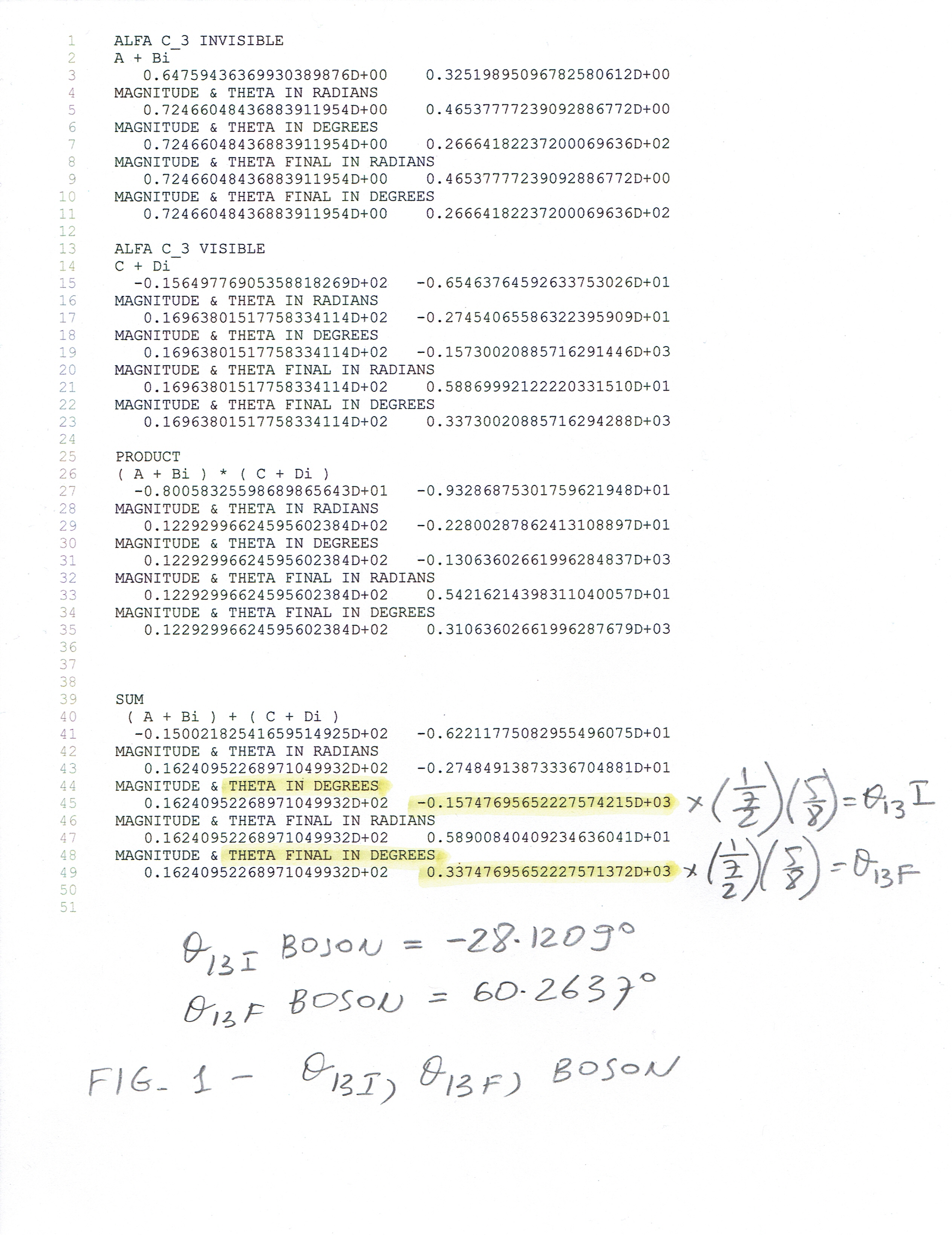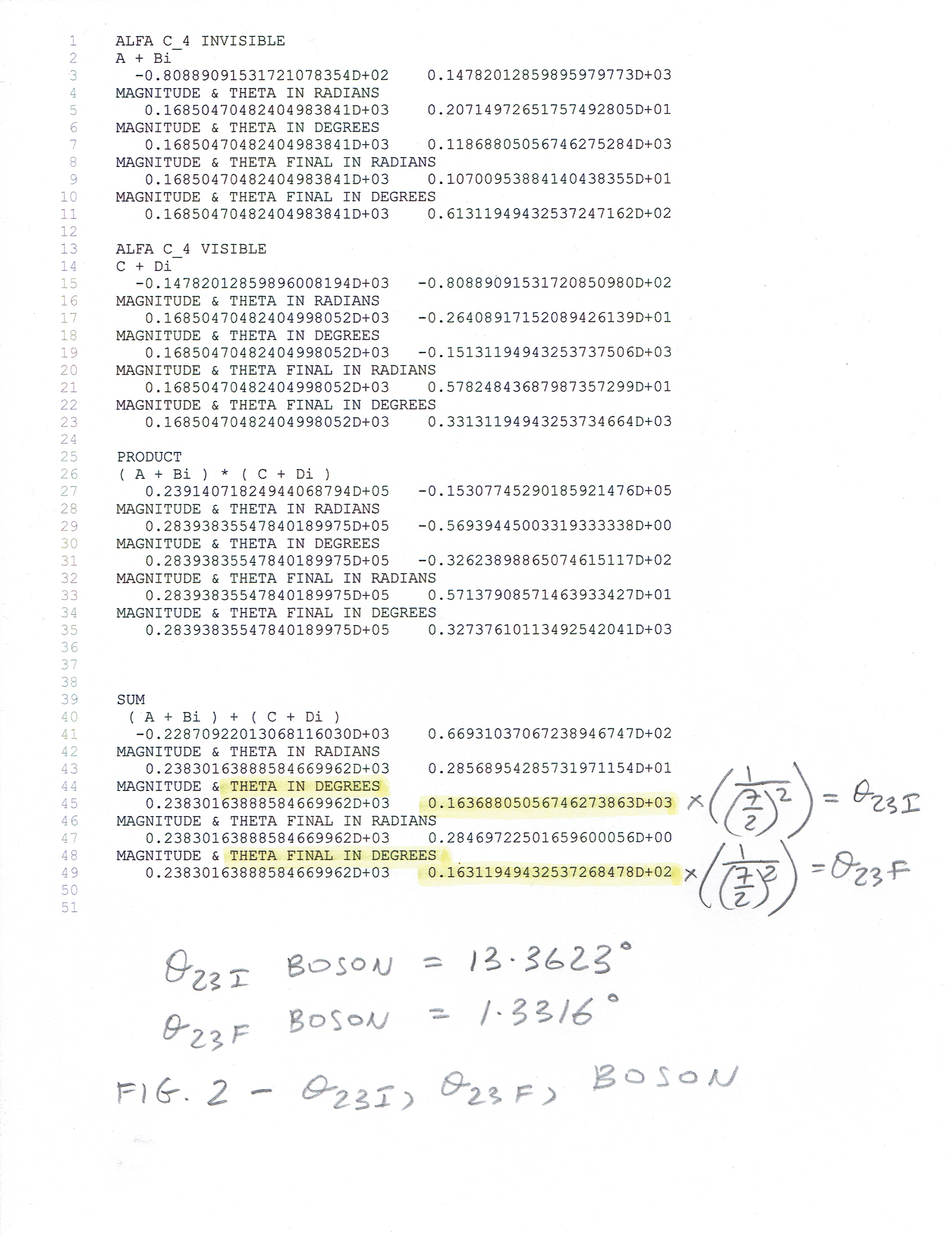St Simeon (107); St Bernadette Soubirous (1879); St Flavian (449)

The Exact Mixing (Oscillation) Angles of Boson - three theta angles. There are Initial and Final values of each angle. Theta(13) Initial is the Weinberg Angle. Two remaining ones are the "educated guesses". Here are the printouts from the FORTRAN programs each for different theta angle.